Go back to  'Circles'

A quadrilateral is cyclic if its vertices are concyclic, that is, if all the four of its vertices lie on a circle. In the following figure, ABCD is a cyclic quadrilateral: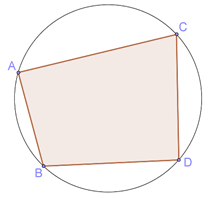Theorem: The opposite angles in a cyclic quadrilateral are supplementary.

An example is shown below: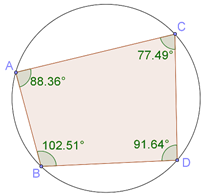Can you prove why this should be true for every cyclic quadrilateral? Also, is the converse of this also true? That is, if both the pairs of opposite angles are supplementary, will it be cyclic?

Let ABCD be a cyclic quadrilateral. Join B and D to the center O of the circle, as shown below: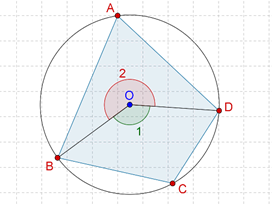Observe that:

$$\angle1=2\times\angle A,\;\;\;\angle2=2\times\angle C$$

è $$\angle 1{\rm{ }} + \angle 2{\rm{ }} = {\rm{ }}{360^0} = {\rm{ }}2(\angle {\rm{A }} + \angle {\rm{C}})$$

è $$\angle {\rm{A }} + \angle {\rm{C }} = {\rm{ }}{180^0}$$

Thus, $$\angle {\rm{A}}$$ and $$\angle {\rm{C}}$$ are supplementary. Similarly, $$\angle {\rm{B}}$$ and $$\angle {\rm{D}}$$ can be shown to be supplementary.

Now, let us prove the converse theorem.

Theorem: If the sum of a pair of opposite angles of a quadrilateral is 180^0, the quadrilateral is cyclic.

Proof: Let us suppose that$$\angle {\rm{A }} + \angle {\rm{C }} = {\rm{ }}{180^0}$$, but the quadrilateral is not cyclic. Draw the circle through A, B and D. By our assumption, it should not pass through C. Let us suppose that this circle intersects CD (or CD extended) at E: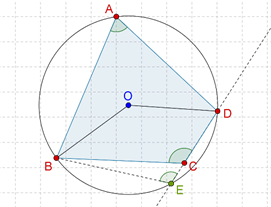Since ABED is cyclic,

$$\angle {\rm{A }} + \angle {\rm{BED }} = {\rm{ }}{180^0}$$

Also

$$\angle {\rm{A }} + \angle {\rm{C }} = {\rm{ }}{180^0}$$

Thus, $$\angle {\rm{BED }} = \angle {\rm{C}}$$ . However, this is not possible, since $$\angle {\rm{C}}$$ (being the exterior angle) must be larger than$$\angle {\rm{BED}}$$ . This can only mean that our supposition that ABCD is not cyclic is incorrect – ABCD is cyclic ! That completes our proof.

Example 1: Let ABCD be a cyclic quadrilateral. Suppose that $$\angle {\rm{DBC }} = {\rm{ }}{55^0}$$ and$$\angle {\rm{BAC }} = {\rm{ }}{45^0}$$. What is the value of $$\angle {\rm{BCD}}$$?

Solution: Consider the following figure: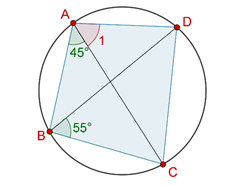We have:

$$\angle 1{\rm{ }} = \angle DBC{\rm{ }} = {\rm{ }}{55^0}$$ (angles in the same segment)

è $$\angle BAD{\rm{ }} = {\rm{ }}{45^0} + {\rm{ }}{55^0} = {\rm{ }}{100^0}$$

Now,

$$\angle BCD{\rm{ }} = {\rm{ }}{180^0}-\angle BAD{\rm{ }}\left( {why?} \right)$$

è $$\angle BCD{\rm{ }} = {\rm{ }}{180^0}-{\rm{ }}{100^0} = {\rm{ }}{80^0}$$

Example 2: Suppose that AB is the diameter of a circle with center O. CD is a chord equal to the radius of the circle. AC and BD are extended to meet at the point E. Find the value of $$\angle {\rm{AEB}}$$ .

Solution: Consider the following figure: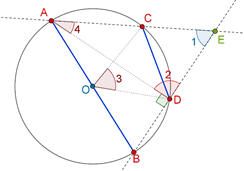We note that $$\Delta {\rm{OCD}}$$ is equilateral (why?), which means that$$\angle 3{\rm{ }} = {\rm{ }}{60^0}$$ . Thus,$$\angle 4{\rm{ }} = {\rm{ }}{30^0}$$ .

Also, note that $$\angle {\rm{ADB}}$$ is an angle in a semi-circle, which means that$$\angle 2{\rm{ }} = {\rm{ }}{90^0}$$ . In$$\Delta {\rm{AED}}$$, we can now easily conclude that$$\angle {\rm{AED }} = \angle 1{\rm{ }} = {\rm{ }}{60^0}$$.

Example 3: Consider the quadrilateral EFGH formed by the internal angle bisectors of a quadrilateral ABCD. Show that EFGH is cyclic.

Solution: Consider the following figure carefully: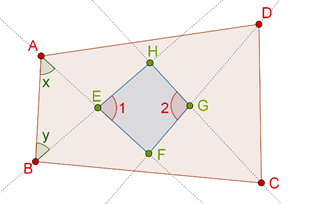The dotted lines represent the angle bisectors. We have to prove that EFGH is a cyclic quadrilateral. If we are able to show that any pair of opposite angles in this quadrilateral is supplementary, our proof would be complete. Thus, we will show that$$\angle 1{\rm{ }} + \angle 2{\rm{ }} = {\rm{ }}{180^0}$$.

Now, consider$$\Delta {\rm{AEB}}$$. Clearly,

$\angle x{\rm{ }} = {\rm{ }}\raise.5ex\hbox{1}\kern-.1em/\kern-.15em\lower.25ex\hbox{2} \angle A,\angle y{\rm{ }} = {\rm{ }}\raise.5ex\hbox{1}\kern-.1em/\kern-.15em\lower.25ex\hbox{2} \angle B$

Thus,

$\angle 1{\rm{ }} = {\rm{ }}{180^0}-{\rm{ }}\raise.5ex\hbox{1}\kern-.1em/\kern-.15em\lower.25ex\hbox{2} \angle A{\rm{ }}-{\rm{ }}\raise.5ex\hbox{1}\kern-.1em/\kern-.15em\lower.25ex\hbox{2} \angle B$

Similarly,

$\angle 2{\rm{ }} = {\rm{ }}{180^0}-{\rm{ }}\raise.5ex\hbox{1}\kern-.1em/\kern-.15em\lower.25ex\hbox{2} \angle C{\rm{ }}-{\rm{ }}\raise.5ex\hbox{1}\kern-.1em/\kern-.15em\lower.25ex\hbox{2} \angle D$

$\angle 1{\rm{ }} + \angle 2{\rm{ }} = {\rm{ }}{360^0}-{\rm{ }}\raise.5ex\hbox{1}\kern-.1em/\kern-.15em\lower.25ex\hbox{2} {\rm{ }}(\angle A{\rm{ }} + \angle B{\rm{ }} + \angle C{\rm{ }} + \angle D)$

But the sum of the four angles of the quadrilateral must be 3600, and so:

$\angle 1{\rm{ }} + \angle 2{\rm{ }} = {\rm{ }}{360^0} - {\rm{ }}{180^0} = {\rm{ }}{180^0}$

This proves the assertion that EFGH is cyclic.

Example 4:. ABCD is a cyclic quadrilateral. The sides AB and DC are produced to meet at X, and the sides AD and BC are produced to meet at Y. Show that the bisectors of $$\angle {\rm{BXC}}$$ and $$\angle {\rm{CYD}}$$ will be perpendicular.

Solution: The solution to this problem might seem to be a bit complicated, so we will present it step-wise. First of all, consider the following figure carefully: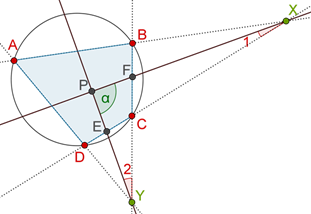The two angle bisectors meet at P (note that P is not the center of the circle). We will show that $$\angle {\rm{XPY }} = \angle \alpha = {\rm{ }}{90^0}$$

Step 1: First, we will find the values of $$\angle 1$$ and$$\angle 2$$. We have:

$\angle {\rm{AXD }} = {\rm{ }}{180^0} - {\rm{ }}(\angle {\rm{A }} + \angle {\rm{D}})$

$\angle {\rm{AYB }} = {\rm{ }}{180^0} - {\rm{ }}(\angle {\rm{A }} + \angle {\rm{B}})$

Thus,

$$\angle 1{\rm{ }} = \frac{1}{2} \angle {\rm{AXD }} = {\rm{ }}{90^0}-\frac{1}{2}(\angle {\rm{A }} + \angle {\rm{D}})$$

$$\angle 2{\rm{ }} = \frac{1}{2}\angle {\rm{AYB }} = {\rm{ }}{90^0}-\frac{1}{2}(\angle {\rm{A }} + \angle {\rm{B}})$$

è $$\angle 1{\rm{ }} + \angle 2{\rm{ }} = {\rm{ }}{180^0}-\angle {\rm{A }}-\frac{1}{2}(\angle {\rm{B }} + \angle {\rm{D}})$$

è $$\angle 1{\rm{ }} + \angle 2{\rm{ }} = \angle {\rm{C }}-\frac{1}{2}\left( {{{180}^0}} \right)$$

è $$\angle 1{\rm{ }} + \angle 2{\rm{ }} = \angle {\rm{C }}-{\rm{ }}{90^0}$$

Step 2: Next, we find the values of ∠PEC and ∠PFC. We have:

$\begin{array}{l} \angle {\rm{PEC }} = {\rm{ }}{180^0}-\angle \alpha-\angle 1\\ \angle {\rm{PFC }} = {\rm{ }}{180^0}-\angle \alpha -\angle 2 \end{array}$

Thus, their sum is

$\angle {\rm{PEC }} + \angle {\rm{PFC }} = {\rm{ }}{360^0}-{\rm{ }}2\angle \alpha -{\rm{ }}(\angle 1{\rm{ }} + \angle 2)$

$= {\rm{ }}{360^0}-{\rm{ }}2\angle \alpha-\angle C{\rm{ }} + {\rm{ }}{90^0}$ $= {\rm{ }}{450^0}-{\rm{ }}2\angle \alpha -\angle C$

Step 3: Now, we consider quadrilateral PECF. The sum of the four interior angles of this quadrilateral must be 3600:

$$\angle\;\alpha\;+\;\angle PEC\;+\;\angle PFC\;+\;\angle C\;=\;360^0$$

è$$\angle \alpha + {\rm{ }}{450^0}-{\rm{ }}2\angle \alpha-\angle {\rm{C }} + \angle {\rm{C }} = {\rm{ }}{360^0}$$

è$$\angle \alpha = {\rm{ }}{90^0}$$

This completes our proof. We have shown that XP and YP are perpendicular.